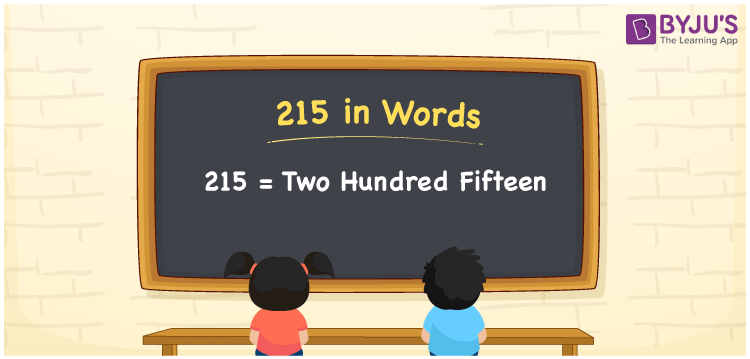# 215 in Words

215 in words can be written as Two Hundred Fifteen. The concepts like counting numbers which are important from primary education are discussed here in brief. For instance, if you spend Rs. 215 in buying footwear, then you can say that “I bought footwear for Two Hundred Fifteen Rupees”. The English alphabet is used to write 215 in words. Students can get a clear knowledge of numbers in words with the help of the study materials given at BYJU’S. The number 215 can be read as “Two Hundred Fifteen” in English.

 215 in words Two Hundred Fifteen Two Hundred Fifteen in Numbers 215

## 215 in English Words## How to Write 215 in Words?

Place value chart and expanded form of 215 can be learnt easily in this section. There are three digits in the number 215. The place value chart for 215 is provided below for reference to help students understand the concepts effectively.

 Hundreds Tens Ones 2 1 5

The expanded form of 215 is provided below:

2 × Hundred + 1 × Ten + 5 × One

= 2 × 100 + 1 × 10 + 5 × 1

= 215

= Two Hundred Fifteen

Hence, 215 in words is written as Two Hundred Fifteen.

215 is a natural number that precedes 216 and succeeds 214.

215 in words – Two Hundred Fifteen

Is 215 an odd number? – Yes

Is 215 an even number? – No

Is 215 a perfect square number? – No

Is 215 a perfect cube number? – No

Is 215 a prime number? – No

Is 215 a composite number? – Yes

## Frequently Asked Questions on 215 in Words

### How can 215 be written in words?

215 can be written as “Two Hundred Fifteen” in words.

### Is 215 an odd number?

No, 215 is an odd number because it is not divisible by 2.

### Write Two Hundred Fifteen in numbers.

Two Hundred Fifteen can be written in numbers as 215.